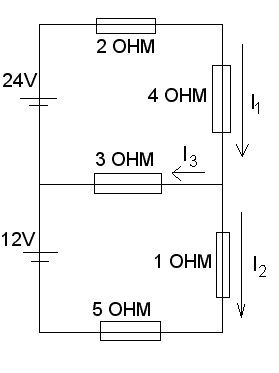# DC current -- Find the 3 currents in this Battery + Resistor circuit

jake jungle

## Homework StatementFind I1 , I2 and I3

I = E / R
I3 = I1 - I2

## The Attempt at a Solution

its the trouble with parallel different emf . I know a formula how to combine them into one but it needs inner resistance . i dont know how to do it with the ideal circuit . The combine emf will go to bigger emf or the sum of them ? and how to find the current then ? please help :(

Mentor

## Homework Statement

View attachment 78584

Find I1 , I2 and I3

I = E / R
I3 = I1 - I2

## The Attempt at a Solution

its the trouble with parallel different emf . I know a formula how to combine them into one but it needs inner resistance . i dont know how to do it with the ideal circuit . The combine emf will go to bigger emf or the sum of them ? and how to find the current then ? please help :(
When in doubt, apply the basics: KVL and KCL. You've already written a correct KCL equation for the circuit. Now what can you do with KVL?

jake jungle
When in doubt, apply the basics: KVL and KCL. You've already written a correct KCL equation for the circuit. Now what can you do with KVL?

so i do it like this :
I1(2+4) +3I3 - 24 = 0 (KVL)
I2(5+1) + 3I3 - 12 = 0 (KVL)
I1 = I2 + I3 (KCL)

and i solve this and got I1 = 3 A , I2 = 1A , I3 = 2A
Am i wrong somewhere cause the answer should be I1=3.5A and I2 =2.5A instead

Mentor
so i do it like this :
I1(2+4) +3I3 - 24 = 0 (KVL)
I2(5+1) + 3I3 - 12 = 0 (KVL)
I1 = I2 + I3 (KCL)

and i solve this and got I1 = 3 A , I2 = 1A , I3 = 2A
Am i wrong somewhere cause the answer should be I1=3.5A and I2 =2.5A instead
Yes, your second KVL equation is incorrect. You didn't take into account the defined direction of i3.

jake jungle
Yes, your second KVL equation is incorrect. You didn't take into account the defined direction of i3.

the direction of i2 and i3 quite confusing
so i reveresed the direction of i2 to apply KVL more easily , therefore i got these equations :
i1(2+4) +3i3 - 24 = 0 (KVL)
i2(5+1) + 3i3 +12 = 0 (KVL)
i3 = i1 + i2 (KCL)

and i got right answer i1 = 3.5A , i2 = -2.5A (due to reversing the direction )

However , if i dont reverse i2 , am i able to use kvl with the second loop ? cause the current is not in the same direction and it confused me .

Mentor
Just leave the currents defined as they are on the diagram. That will make sure that the signs of the currents that you obtain will agree with their definitions on the circuit diagram.

When you do your "KVL walk" around the loop, if you pass though a resistor in the same direction as its current then you have a potential drop. If you pass through a resistor against the current flow then you have a potential rise. Write the signs of the terms in your KVL equiations accordingly.

•jake jungle
jake jungle
OK I got it , thanks alot